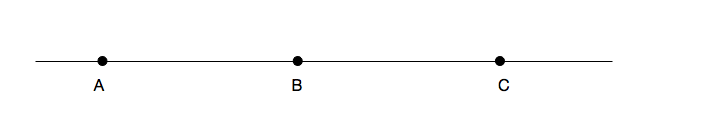Geometry

# Distance between Two Points

Given the line segment $\overline{PQ}$ with $P=(12,11)$ and $Q=(8,5)$, the length of the line segment is $a$.

What is $a^2$?The points $A,B,$ and $C$ lie on a straight line such that the distance from $A$ to $B$ is 4 inches, and the distance from $B$ to $C$ is 5 inches. An ant crawled along the straight line from $A$ to $C$, and then back to $B$. What is the total distance (in inches) that the ant travelled?

If $A=(1,a)$, $B=(4,2)$, and the distance between $A$ and $B$ is 5, what is the positive value of $a?$

What is the distance between $(4, 8 )$ and the origin?

Let $a$ be a point on a number line such that its distance from the point $3$ on the same number line is $9.$ What are the possible values of $a ?$

×